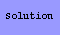Triangulation Puzzles

# 1.

Cut a unit square into 5 triangles, all of which have area less than .21.# 2.

Cut a square into 5 triangles whose areas are in geometric progression.# 3.

Cut a square into 5 isoceles triangles, no two of which are similar.
(solution by Evan Whitney)# 4.

Cut a square into 7 isoceles right triangles, no two of which are congruent.
(second solution by Bob Wainwright)# 5.

Cut a square into 8 right triangles, all having one leg twice
as long as the other, no two of which are congruent.# 6.

Cut a square into 8 acute triangles.
(solution by David Epstein)# 7.

Cut a unit square into 8 triangles with sides no larger than 5/8.# 8.

Pack 7 equilateral triangles with side 1/2 into a unit square.